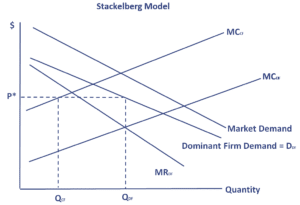# Stackelberg model

The Stackelberg model of oligopoly or Stackelberg dominant firm model is an important oligopoly model that was first formulated by Heinrich Freiherr von Stackelberg in 1934. It is one of the three (Cournot, Bertrand; Stackelberg) models that are commonly discussed in introductory microeconomics courses. While the Stackelberg model was originally formulated as a duopoly model, nowadays it is often discussed as a model with one dominant firms and a large number of smaller firms.

The Stackelberg leadership model is a sequential model, which means that the dominant firm first sets the price, which is then used by the other firms to determine their optimal production. This is different from the Cournot duopoly, where both companies set their production simultaneously.

# Stackelberg duopoly model definition

In the Stackelberg model, we have a single firm that has a significantly large market share. The large market share can be assumed to be the result of greater scale and thus lower costs. The price in the market depends on the marginal cost of this dominant firm. In particular, the dominant firm will set the price such that marginal cost (MC) equals marginal revenue (MR). This level of output then determines the market price.

It is important to note that the dominant firm believes that the quantity supplied by the other smaller companies decreases when the price goes down. Thus, the dominant firm’s demand for its product is related to the market demand curve in the way shown in the below figure.The dominant firm’s demand curve should be used to determine the price at which the dominant firm will sell its product. The other firms will then maximize profits by using this price to set their own output. This is the price for which the market price equals their marginal cost.

A price decrease by one of the smaller competitive firms will lead to a drop in the price by the dominant firm. This price decrease will then lead the smaller firms to decrease output or exit the industry. The long-run impact of one the small firms price decrease will be an increase in the market share of the small firms as they are forced to exit the market. Thus, the competitive firms have no incentive to lower the price. If the competitive firms engage in such behavior, they will hurt themselves.

## Summary

The Stackelberg price competition model is an important model that captures the game theory behavior of a market where one company has a dominant position. We discussed that this company tends to set the price based on its marginal cost. The other companies then take this price as given and set the own output.